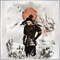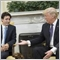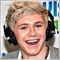• 概述
• 评论 (3)
• 评论 (10)
• 新特性

# MACM Indicator

If MACD means Moving Average Convergence Divergence.

MACM means Moving Average Currency Meter.

*IMPORTANT NOTE : MACM is a very intricate indicator which calculates at most of252 Signals (28 pairs x 9 Time frames). Due to its complexity, a 2nd indicator (FREE INDICATOR) has to be made for it to work accurately. Download the MACM Scanner here. https://bit.ly/2T006uw

MACM is a revolutionary indicator with new sets of calculations never seen before.By it's name. MACM is a combination of MA and CM --- Moving Average and Currency Meter. It is basically a Moving Average of Currency Meter.

To give you an idea to this very NEW concept, MACM is like a moving average but with the algorithm of moving average and currency strength of 28 pairs put it one!

MACM have 3 basic features.

Click Button 1

• Currency Directions on each Pair-- 7 Signals on 8 Currencies = 56 Signals for 28 Pairs..
• Signal of a currency on 9 Time Frames = +9 Signals
• 65 Signals Total

Click Button 2

• Signal of 28 Pairs by 9 Timeframes = 252 Signals in a single chart.

Click Button 3

• Hide Signals. This would only shows the maximum line being drawn..

MACM Parameters :

• MA Period - Moving Average Period
• MACM Period - Moving Average Currency Meter Period
• Method - Simple, Exponential, Smoother Linear or Smoothed
• Moving Average Shift - Shift of prices
• Price - Type of Price
• <<<<<<<<
• Font - Font Size
• <<<<<<<<<
• MACM Trend Line (Candles) -
• Candles - The Number of Candles where the trend line is drawn. Default is 1000 to minimize delay or lag on your chart.
• <<<<<<<<<<
• Button horizontal distance - Parameter to edit the placement of button horizontally
• Button vertical distance - Parameter to edit the placement of button vertically
• <<<<<<<<<<
• UP Trend - Color Up Trend
• Down Trend - Color of Down Trend
• Pairs and Time Frames - Color of Alert and TimeFrames
• <<<<<<<<<<
• Moving Average line - By default, Color of MA Line is Red
• Moving Average Currency Meter Line - By default, Color of MACM Line is Yellow
• <<<<<<<<<<
• Alert User - You can choose either by Time Interval Alert or by Change of Signal Aler52
2020.02.06 17:19

Indicator is legit AF! Easy to use75
2020.01.08 15:33

Good indicator. Author wont force you to give 5-star for Free Scanner. Bravooo!28
2020.01.06 12:31

So good so far. It's very good to see all the movements of the pairs too on all timeframes. Especially this MACM concept. Very Satisfied!

Parameters re-arranged